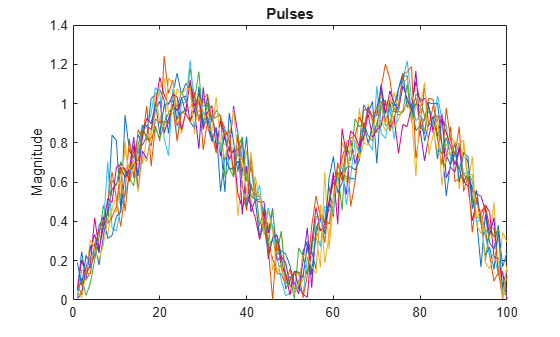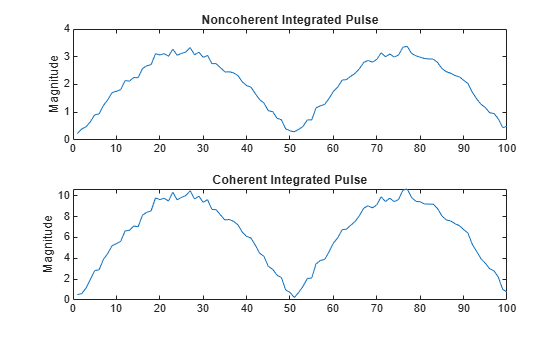# pulsint

Pulse integration

## Syntax

``Y = pulsint(X)``
``Y = pulsint(X,METHOD)``

## Description

example

````Y = pulsint(X)` performs video (noncoherent) integration of the pulses in `X` and returns the integrated output in `Y`. Each column of `X` is one pulse.```

example

````Y = pulsint(X,METHOD)` performs pulse integration using the specified method. `METHOD` is `"coherent"` or `"noncoherent"`.```

## Examples

collapse all

Generate ten pulses of a sinusoid with added Gaussian white noise. Plot the pulse magnitudes.

```npulse = 10; x = repmat(sin(2*pi*(0:99)'/100),1,npulse) + 0.1*randn(100,npulse); plot(abs(x)) ylabel("Magnitude") title("Pulses")```Perform noncoherent integration of the pulses. Repeat the computation, but now perform coherent integration. Plot the magnitudes of the integrated pulses

```yn = pulsint(x); subplot(2,1,1) plot(abs(yn)) ylabel("Magnitude") title("Noncoherent Integrated Pulse") yc = pulsint(x,"coherent"); subplot(2,1,2) plot(abs(yc)) ylabel("Magnitude") title("Coherent Integrated Pulse")```## Input Arguments

collapse all

Pulse input data, specified as a matrix. Each column of `X` is one pulse.

Example: ```sin(pi./[32 32].*(0:2047)')+randn(2048,2)/10```

Data Types: `double`

Pulse integration method, specified as `"coherent"` or `"noncoherent"`. `METHOD` is the method used to integrate the pulses in the columns of `X`. The values are not case sensitive.

Data Types: `char` | `string`

## Output Arguments

collapse all

Integrated pulse, returned as an N-by-1 column vector, where N is the number of rows in the input `X`.

collapse all

### Coherent Integration

Let Xij denote the (i,j)-th entry of an M-by-N matrix of pulses X.

The coherent integration of the pulses in X is:

`${Y}_{i}=\sum _{j=1}^{N}{X}_{ij}$`

### Noncoherent (video) Integration

Let Xij denote the (i,j)-th entry of an M-by-N matrix of pulses X.

The noncoherent (video) integration of the pulses in X is:

`${Y}_{i}=\sqrt{\sum _{j=1}^{N}|{X}_{ij}{|}^{2}}$`

## Version History

Introduced in R2011a